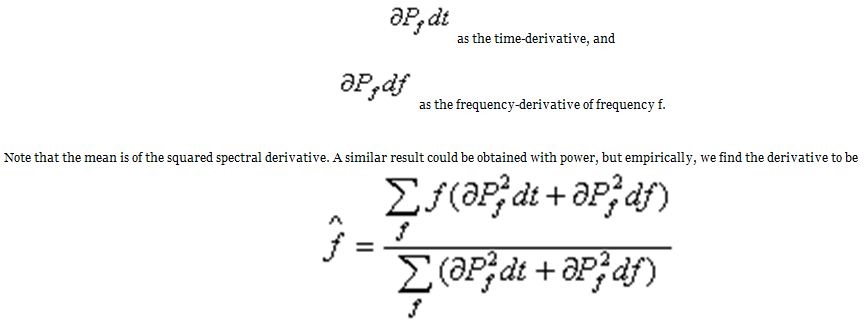# Mean frequency

 < PREVIOUS: Pitch related measures > NEXT: Peak frequency Mean frequency is a pitch measure, that assesses the center of the distribution of power across frequencies. Here is an example of mean frequency estimates in a thrush nightingale song: -As indicated by the purple curve, the mean frequency provides a smooth estimate of the concentration of spectral power. This estimate is often (but not always) superior to peak frequency, which might 'jump' from one harmonic to the next. Formal definition: Mean frequency is an estimate of the central tendency of the derivative power distributions. Derivative power is calculated by adding the partial derivatives of powers, namely:In contrast to peak-frequency, mean frequency provides a smooth estimate of the center of derivative power. In contrast to peak frequency, mean-frequency does not ‘stick’ to any frequency trace, as shown in the example above.